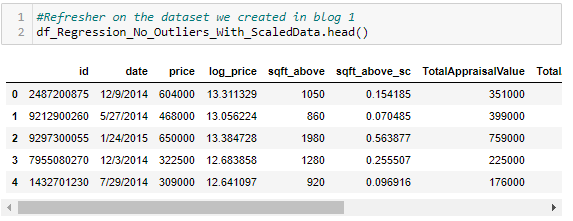# Introduction

Using the past to predict the future! Say hello to part 2 of 3 in this series on regression modeling with python! In blog 1, I covered the important processing steps prior to creating a linear regression model. In this blog I build from that foundation by creating the actual regression model along with demonstrating how to reuse the model with new data. In blog 3 I will cover how to check for the post-linear model creation assumptions.King County Data + Scraped Data From King County Tax Assessors

# 1.Creating Our Model

With cleaned and assumption adhering data in hand, our first step is to separate our target from our features.

`# Import appropriate Statsmodel Librariesimport statsmodels.api as sm# Separate Our Variables# Get our Target Variable – Actual Price Reacheddf_Regresion_Target = df_Regression_No_Outliers_With_ScaledData['log_price']#Get our Features - Remove any columns not used to predict our target.df_Regresion_Features = df_Regression_No_Outliers_With_ScaledData.drop(columns=['id', 'date', 'price','log_price',‘sqft_above',  'TotalAppraisalValue'])# Use Features to create y interceptpredictors_int = sm.add_constant(df_Regresion_Features)# Feed Features and intercept to Model Method for Model creationmodel = sm.OLS(df_Regresion_Target,predictors_int).fit()#View modelmodel.summary()`
`#Run Predictions using the modeldf_predictedPrice = model.predict(predictors_int)#Create Dataframe From Predictionsf_predictions = pd.DataFrame(df_predictedPrice, columns=["log_price_Predicted"])df_predictions["price_Predicted"] = np.exp(df_predictions["log_price_Predicted"])df_predictions["log_Residuals"] = model.resid#Combine Predictions with Original datafromedf_predictions = df_predictions.reset_index(drop=True)df_Regression_No_Outliers_With_ScaledData = df_Regression_No_Outliers_With_ScaledData.reset_index(drop=True)df_Regression_No_Outliers_With_ScaledData = pd.concat([df_Regression_No_Outliers_With_ScaledData, df_predictions], axis=1)df_Regression_No_Outliers_With_ScaledData['price'] = df_Regression_No_Outliers_With_ScaledData['price'].astype(float)df_Regression_No_Outliers_With_ScaledData["price_Residuals"] =  df_Regression_No_Outliers_With_ScaledData.apply(lambda x: x['price_Predicted'] - x['price'], axis=1)#Reorder columns, put prices next to each otherdf_Regression_No_Outliers_With_ScaledData = df_Regression_No_Outliers_With_ScaledData[['id', 'date', 'price', 'price_Predicted', 'price_Residuals', 'log_price', 'log_price_Predicted','log_Residuals','sqft_above', 'Sch_d_Top15','Sch_d_Top30', 'Sch_d_Top60', 'TotalAppraisalValue', 'sqft_above_sc','TotalAppraisalValue_sc',]]#Display Resultsdf_Regression_No_Outliers_With_ScaledData.head()#Run Predictions using the modeldf_predictedPrice = model.predict(predictors_int)#Create Dataframe From Predictionsdf_predictions = pd.DataFrame(df_predictedPrice, columns=["log_price_Predicted"])df_predictions["price_Predicted"] = df_predictions.apply(lambda x: np.exp(x['log_price_Predicted']), axis=1)df_predictions["log_Residuals"] = model.resid#Combine Predictions with Original datafrome, create Residuals column by substracting Actual From Predicteddf_predictions = df_predictions.reset_index(drop=True)df_Regression_No_Outliers_With_ScaledData = df_Regression_No_Outliers_With_ScaledData.reset_index(drop=True)df_Regression_No_Outliers_With_ScaledData = pd.concat([df_Regression_No_Outliers_With_ScaledData, df_predictions], axis=1)df_Regression_No_Outliers_With_ScaledData['price'] = df_Regression_No_Outliers_With_ScaledData['price'].astype(float)df_Regression_No_Outliers_With_ScaledData["price_Residuals"] =  df_Regression_No_Outliers_With_ScaledData.apply(lambda x: x['price_Predicted'] - x['price'], axis=1)df_Regression_No_Outliers_With_ScaledData["price_Residuals_abs"] =  abs(df_Regression_No_Outliers_With_ScaledData["price_Residuals"])#Reorder columns, put prices next to each otherdf_Regression_No_Outliers_With_ScaledData = df_Regression_No_Outliers_With_ScaledData[['id', 'date', 'price', 'price_Predicted', 'price_Residuals','price_Residuals_abs','log_price','log_price_Predicted','log_Residuals','sqft_above', 'Sch_d_Top15','Sch_d_Top30', 'Sch_d_Top60', 'TotalAppraisalValue','sqft_above_sc','TotalAppraisalValue_sc',]]#Display Resultsdf_Regression_No_Outliers_With_ScaledData.head()`
`#Graph our residuals against our predictions, this will give us a sense if our model is off for certain priced homesplt.scatter(df_predictions["price_Predicted"], df_Regression_No_Outliers_With_ScaledData["price"])plt.xlabel("Predicted Price")]plt.xticks(ticks=(200000, 400000, 700000, 900000),labels= ('\$200k', '\$400k', '\$700k', '\$900k'))plt.ylabel("Residual")plt.yticks(ticks=(200000, 400000, 700000, 900000),labels= ('\$200k', '\$400k', '\$700k', '\$900k'))plt.plot([200000, 900000], [200000, 900000], color = 'red', linewidth = 2)plt.show()`
`#Graph our residuals against our predictions, this will give us a sense if our modle is off for certain priced homesplt.scatter(df_predictions["price_Predicted"], df_Regression_No_Outliers_With_ScaledData["price_Residuals"])# plt.plot(Model1.predict(df_X_train), [0 for i in range(len(df_X_train))]);plt.xlabel("Predicted Price")plt.xticks(ticks=(300000, 500000, 700000, 900000, 1200000),labels= ('\$300k', '\$500k', '\$700k', '\$900k','\$1.2M'))plt.ylabel("Residual")plt.yticks(ticks=(-300000, -100000, 0, 300000, 500000),labels= ('\$-300k', '\$-100k', '\$0k', '\$300k','\$500k'))plt.show()`

# 2. Using Your Model Again

What if we want to make new predictions? Do we have to add that new house into our original data source as another row and start over from step 1? Thankfully, no. All you need to do is the following:

`# Create the average home used the average from each featured = {'a_price'[df_Regression_No_Outliers_With_ScaledData['price'].mean()]}df_The_Average_Home = pd.DataFrame(data=d)df_The_Average_Home['a_log_price'] = df_Regression_No_Outliers_With_ScaledData['log_price'].mean()df_The_Average_Home['a_sqft_above'] = df_Regression_No_Outliers_With_ScaledData['sqft_above'].mean()df_The_Average_Home['a_Sch_d_Top15'] = 0df_The_Average_Home['a_Sch_d_Top30'] = 0df_The_Average_Home['a_Sch_d_Top60'] = 1df_The_Average_Home['a_TotalAppraisalValue'] = df_Regression_No_Outliers_With_ScaledData['TotalAppraisalValue'].mean()df_The_Average_Home['a_sqft_above_sc'] = df_Regression_No_Outliers_With_ScaledData['sqft_above_sc'].mean()df_The_Average_Home['a_TotalAppraisalValue_sc'] = df_Regression_No_Outliers_With_ScaledData['TotalAppraisalValue_sc'].mean()# Display the average homedf_The_Average_Home.head()`
`# Create dataframe to feed into scaler, make sure not to feed scaler your categorical datadf_New_Data_To_Be_Scaled = df_The_Average_Home[["a_sqft_above","a_TotalAppraisalValue"]]# Feed data into scales using the .transform() method vs. .fit_transform()Newly_scaled_Data = scaler.transform(df_New_Data_To_Be_Scaled)# Create dataframe from newly scaled valuesdf_Newly_scaled_Data = pd.DataFrame(Newly_scaled_Data, columns=["sqft_above_sc","TotalAppraisalValue_sc"])df_Newly_scaled_Data = df_Newly_scaled_Data.reset_index(drop=True)# Get caegorical values to feed into new predictiondf_Average_Categoricals = df_The_Average_Home[['a_Sch_d_Top15', 'a_Sch_d_Top30','a_Sch_d_Top60']]#Bring two sets of data together prior to feeding dataframedf_Average_Categoricals = df_Average_Categoricals.reset_index(drop=True)df_New_Row_For_Prediction = pd.concat([df_Newly_scaled_Data, df_Average_Categoricals], axis=1)#add contanct in position 1df_New_Row_For_Prediction.insert (0, "constant", 1)# Use Features to create interceptnew_predictors_int = sm.add_constant(df_New_Row_For_Prediction)Newpredicted_value_log = model.predict(df_New_Row_For_Prediction)Newpredicted_value = np.exp(Newvalue_log)Newpredicted_value`

# Conclusion & Preview of Blog 3

Above I covered the steps to create a model using Statsmodel. I also demonstrated how you can reuse your model with new data. In blog 1 I demonstrated some of the important processing steps required prior to model creation. In blog 3 I will finish my introduction to linear regression modeling by reviewing how to check for the remaining two assumptions. I look forward to seeing you soon!

--

--